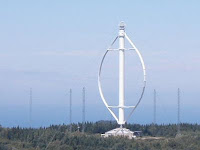“Be kind, for everyone you meet is fighting a hard battle” - Often attributed to Plato but likely from Ian McLaren (pseudonym of Reverend John Watson)

## Friday, November 19, 2010

### What can the wind do?

After my post regarding the wind turbines atop Houston's Hess tower, I thought a very brief and very elementary primer on wind power might be in order. Moving air posesses kinetic energy in accordance with E=1/2*m*v^2. This energy can be harnessed to generate electricity. So how much energy is this?

Let's look at the turbines from the Hess Tower that I discussed in the post.  Here, each turbine intercepts an area of 8.25 m^2. Let's let s be the wind speed (since the turbines have a vertical axis the wind direction doesn't matter, so we don't need the vector quantity) in m/s (meters per second). Then, in one second, each turbine will intercept a volume V of 8.25*s m^3 (meters cubed). Assuming air has a density, d, of 1.16 kg/m^3, the mass,m, of air intercepted in a second is V*d=8.25*s*1.16 kg. This mass, moving at a speed of s m/s has a kinetic energy, E, of 0.5*m^s^2 or 0.5*8.25*s*1.16*s^2.

One thing to note before moving on is that s is present twice - once to the first power and once squared - meaning that the available energy PER SECOND (remember, we are talking about the amount of energy available in one second's worth of wind) is proportional to the cube of the wind velocity. Now, the total available energy per second in the wind is otherwise known as power. In other words, this number is the power that would be produced at a given wind speed, s, if 100% of it could be converted to electrical energy. Of course it can't, and we'll get to that later.

So, where are we? For a speed, s, the kinetic energy, E, per second, or power, P, in watts passing through one of the Hess turbines is 0.5*8.25*1.16*s^3 or, P=4.785*s^3 watts. Like a good physicist, let's check the units: 0.5 is dimensionless; 8.25 is m^2; 1.16 is kg*m^(-3); s is m*s^(-1) but it's cubed so that factor has units m^3*s^(-3). Thus, we have: for m, m^2*m^(-3)*m^3 or m^2; kg is merely kg^1; for s we have  s^(-3). The total is kg*m^2*s^(-3). Now, power is energy per second, or force * distance/second, or (mass*distance/time^2)*distance/second. This is kg^1*m*s^(-2)*m*s^(-1) or kg*m^2*s(-3). The units check. For ANY equation relating physical quantities, the units on each side MUST match, this is a necessary (but not sufficient) condition for the equation to be valid.

Let's stick a number in and see what we get. A 15 m.p.h. wind is fairly robust, how much power is available for the turbine's conversion to electricity? 15 m.p.h. is 6.71 m/s, the total power (through an area the size of a single Hess turbine) is 4.785*6.71^3 or 1,443 watts. If it all could be converted to electricity, it would light a little more than 14 100 watt light bulbs.Of course it can't all be converted. One excellent intuitive way of understanding this is that the kinetic energy comes from the speed, to extract it all we would need to bring the wind to a complete stop, requiring a solid wall at the "turbine" location, something that obviously cannot work. Albert Betz was the first to calculate the absolute theoretical maximum energy that could ideally be extracted from wind. This is called, appropriately enough, the Betz Limit, and it's 59.3%.

Now a real turbine will not approach this limit, and horizontal axis wind turbines, at least in steady wind, are more efficient than vertical axis wind turbines like those at Hess Tower. From the data available at the
Cleanfield Energy site (the manufacturer of the Hess turbines) I estimate that the claim is that the turbines are about 37% efficient.

I've included a chart showing the total power in wind, as well as the Betz Limit and the power at 37% efficiency for wind from 0 to 30 m.p.h. The actual numbers apply specifically to a single Cleanfield turbine like the ones on the Hess Tower, but the shapes apply to all wind turbines, and keep in mind that the turbines typically don't turn at all until the wind speed reaches somewhere around 8 or 10 m.p.h. (Click to enbiggen).

Here's a graph showing the Betz Limit power in wind for speeds from 2.5 m/s to 20 m/s (about 5.6 m.p.h. to 44.7 m.p.h.) and turbine area of 5 m^2 to 25 m^2. Note the extreme dependence on turbine size and, especially, wind speed.

Ed Davies said...

...each turbine intercepts an area of 8.25 ft^2

...intercept a volume V of 8.25*s m^3

I suspect that the ft^2 in the first quoted text should be m^2. 8.25 ft^2 would be tiny.

¹²³⁴

King of the Road said...

You're right Ed, thanks for catching it. I've corrected the typo. I did use meters in al the calculations.